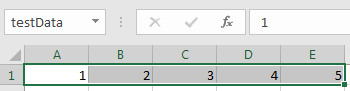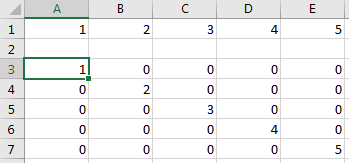## Create Diagonal Matrix Using VBA Macro

This example shows how to execute Spreadsheet Link™ functions to export a named range in the worksheet to MATLAB® and create a diagonal matrix using a Microsoft® Excel® VBA macro.

The example assumes that MATLAB is running after Microsoft Excel opens. For details, see Start and Stop Spreadsheet Link and MATLAB.

In a worksheet, enter the numbers 1 through 5 into the range of cells from `A1` through `E1`. Define the name `testData` for this range of cells and select it. For instructions, see Excel Help and enter the search term: define and use names in formulas.

The named range `testData` appears in the Name Box.On the Developer tab in Microsoft Excel, click . The Visual Basic® Editor window opens.

Insert a new module and create a diagonal matrix from the data in `testData`. To insert the module, select Insert > Module. In the Code section, enter this VBA code that contains a macro named `Diagonal`.

```Sub Diagonal() MLPutRanges MLEvalString "b = diag(testData);" MLGetMatrix "b", "A3" MatlabRequest End Sub```

The `Diagonal` macro exports the named range into the MATLAB variable `testData` using the `MLPutRanges` function. Then, the macro uses the `MLEvalString` function to execute MATLAB code. The MATLAB code creates a diagonal matrix from the data in `testData` using the `diag` function. The code assigns the diagonal matrix to the MATLAB variable `b`. Then, the macro uses the `MLGetMatrix` function to import the diagonal matrix into the worksheet.

Copy and paste the code into the Visual Basic Editor from the HTML version of the documentation.

For details about working with modules, see Excel Help.

Run the macro by clicking . For details about running macros, see Excel Help.

The diagonal matrix displays in the worksheet cells `A3` through `E7`.